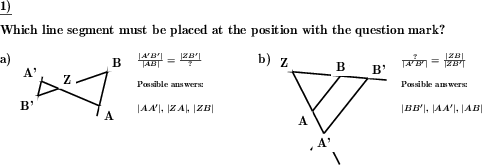Custom math worksheets at your fingertips# Details for problem "Add missing line segment name for intercept theorem"

Quickname: 2896

Junior High School, Middle School, High School.

## Summary

In a statement of the intercept theorems the missing line segment name has to be inserted.

## Example## Description

A labeled drawing to illustrate the intercept theorems is shown. For this purpose, an equation is presented with respect to the ratios of side segment lengths to each other, which represents the application of a set of rays. One of the line's name has been replaced by a question mark ?.

The task is to identify the name of the fourth line, which is in the equation where the question mark is. If desired, a selection of line segments can be presented as possible answers; one of them is correct, the others lead to false statements.

Which theorems are tested is configurable. The figure is presented according to the queried ray-set: For the first and second theorem the figure has two straight lines or rays, for the third theorem three. The figure can be drawn in V-shape, X-shape, or in a random selection of both variants.

The inscription of the points can be optionally

• fixed to Z for the intersection of the lines, A and A' for the points on one line and B and B' for the points on the other line.
• random for the intersection, and random pairs of X and X' for both lines.
• completely random, each point is labeled individually.

The size of the drawing can be selected in several steps. The number of problems can also be configured.

Download free printable worksheets for this math problem here. The worksheet contains the problems only, the solution sheet includes the answers. Just click on the respective link.

•Worksheet 1Solution sheet with answers
•Worksheet 2Solution sheet with answers
•Worksheet 3Solution sheet with answers

If you can not see the solution sheets for download, they may be filtered out by an ad blocker that you may have installed. If this is the case, please allow ads for this page and reload the page. The solution sheets will then reappear.

• Do these sample worksheets do not really fit?
• Do you need more math worksheets, with a different level of difficulty?
• Would you like to combine different problems on a worksheet and adjust them to your needs?
• As a teacher, you can put together your own worksheets using the automatically generated math problems provided.
With a free initial credit, you can start creating your own math worksheets in a few minutes.

You can try it for free! Register here, to create custom worksheets now!

## Customization options for this problem

Parameter
Possible values
Number of problems
1, 2, 3, 4
Size of drawing
small, medium, large
Point names
Z,A,A',B,B', Pairs with ', All different
Theorems covered
First, second, third, First, Second, Third, First & second, First & third, Second & third
Type of drawing
V or X, V, X
Multiple choice style
Yes, No

## Similar problems

Remark
Description
Concrete application of the intercept theorems
In application of the intercept theorem the fourth line segment length is to be calculated from three given lengths.
Determine whether a ratio equation, i.e. intercept theorem-like statement is correct.
Correct statements on the intercept theorems must be identified and marked.